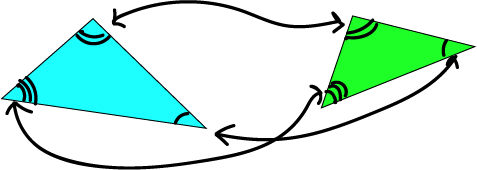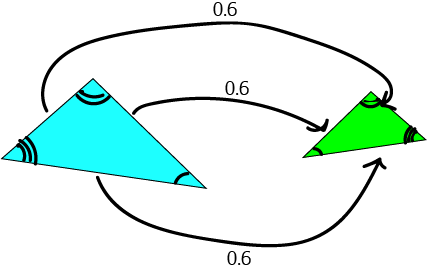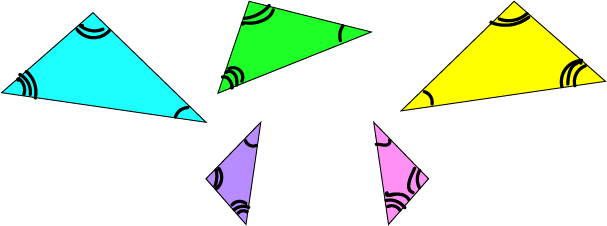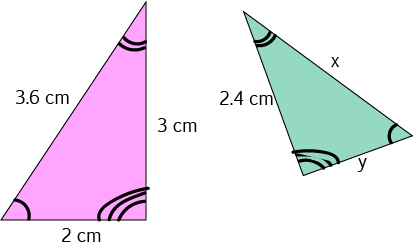# Similar Triangles

We've talked about similar objects before. Similar objects look the same, but they might have different sizes. You can turn a geometric shape into a similar shape by performing one or more of the transformations: rotation, reflection, translation and enlargement.

If two triangles are similar then

• They have three pairs of equal angles• They have three pairs of corresponding sides, with lengths in the same ratio.Notice that the pairs of corresponding sides are opposite the corresponding angles in all cases. For example, the sides opposite the angles marked with three arcs form a pair of corresponding sides.

These triangles are all similar (I've marked the angles that are equal with the same numbers of arcs: 1, 2 or 3)## Corresponding Sides

If we know that a pair of triangles is similar, then we know that their three pairs of corresponding sides are always in the same ratio.

For example the pink and green triangles below are similar:All three pairs of corresponding sides are in the ratio $3:2.4$, or $5:4$.

The lengths 3 cm and 2.4 cm are corresponding: they are both opposite the angle with one arc.

The lengths 3.6 cm and x are corresponding: they are both opposite the angle with three arcs.

The lengths 2 cm and y are corresponding: they are both opposite the angle with two arcs.

## Calculating the Lengths of Corresponding Sides of Similar Triangles

This is easy: just find the ratio, and then use it to find the unknown side-lengths.

### Example:

Find the unknown side lengths for the similar triangles in the picture belowSolution:

As the pink and green triangles are similar, each pair of corresponding sides is in the same ratio. First, find the ratio:

The sides of lengths 2.4 cm in the green triangle and 3 cm in the pink triangle are corresponding (both are opposite the angle marked with one arc).

Therefore, the ratio of the lengths of the corresponding sides of the two triangles is $\dfrac{2.4}{3} = \dfrac{4}{5}$.

Now we know that each side of the green triangle has length equal to $\dfrac{4}{5}$ of the length of the corresponding side of the pink triangle.

Side $x$ corresponds to the side of length 3.6 cm in the pink triangle (both are opposite the angle with 3 arcs). Therefore,

$x = \dfrac{4}{5} \times 3.6 = 2.88$ cm.

Side $y$ corresponds to the side of length 2 cm in the pink triangle (both are opposite the angle with 2 arcs). Therefore,

$y = \dfrac{4}{5} \times 2 = 1.6$ cm.

Now you know that you can use similar triangles to calculate unknown lengths of sides of triangles, you won't be suprised to hear that you can use similar triangles and the lengths of shadows to calculate the heights of trees or buildings. You can also use them to calculate unknown distances. Police radar uses similar triangles to detect speeding cars.

Of course, I haven't told you how to prove that two triangles are similar. There are a number of theorems that can help you to do this. If you want to find out more, have a read of the article on checking whether two triangles are similar.

### Description

In these chapters you will learn about plane geometry topics such as

• Area (Irregular polygons, plane shapes etc)
• Perimeter
• Conic sections (Circle, Ellipse, Hyperbola etc)
• Polygons (Congruent, polygons, similar, triangles etc)
• Transformations and symmetry (Reflection, symmetry, transformations etc)

etc

Even though these chapters are marked for Year 10 or higher students, several topics are for students in Year 8 or higher

### Audience

Year 10 or higher, suitable for Year 8 + students as well.

### Learning Objectives

You must be logged in as Student to ask a Question.